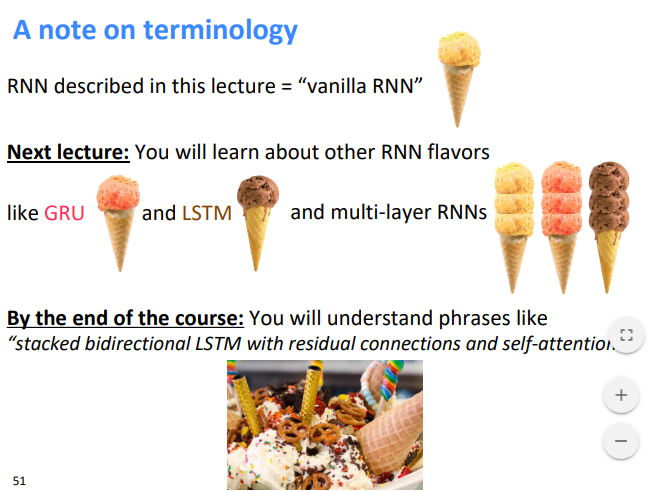# [XCS224N] Lecture 6 – Language Models and RNNs

## Language Modeling

Language Modeling: task of predicting what words come next.

• i.e.compute the conditional probability distribution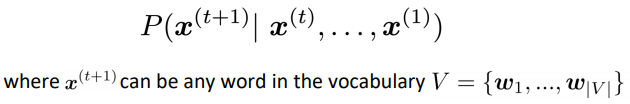• a language model can also be viewed as a system to give probability to a piece of text.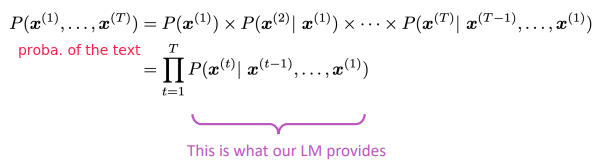## n-gram Language Models

n-gram Language Model: pre-deep learning solution for language modelling.

idea: Collect statistics on frequency of n-grams, and use these to predict next word

(assumption: current word only dep on previous n-1 words)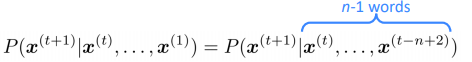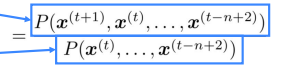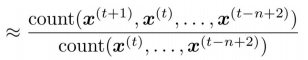sparsity problem:

• some ngrams never appear in training data
• partial solution: smoothing, i.e. add small delta to all words in Vocab
• or the context n-1 gram is never in training data
• partial solution: back-off, i.e. just condition on the n-2 gram
• storage problem: need to store counts for all ngrams

language model to generate text: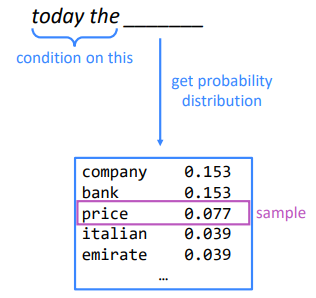## Neural Language Models

window-based neural model: use a fixed window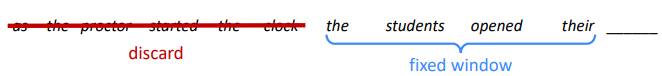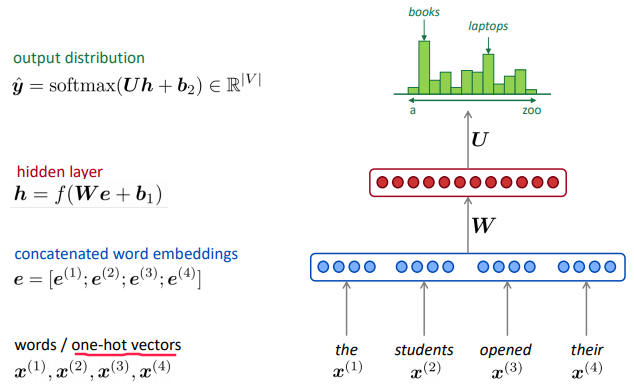⇒ compared to ngram model:

• no sparsity problem
• no storage problem

remaining problems:

• window too small
• words at different positions use different weights, no symmetry

⇒ need a NN model that can process any length input

## RNN Overview

A new family of NN:

• hidden state = function of prev hidden state and curr input vector
• apply the same weights `W` repeatedly.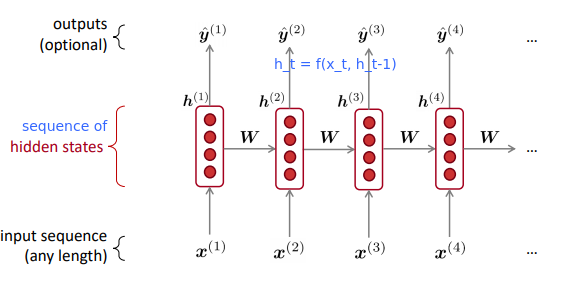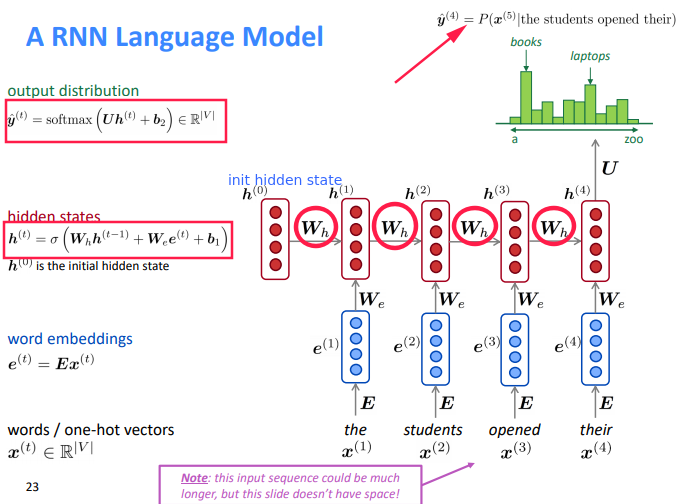pros:

• any length input
• Computation for step `t` can (in theory) use information from many steps back
• Model size doesn’t increase for longer input
• symmetry in how inputs are processed: apply the same weights Wh and We

cons:

• slow
• difficult to access information from many steps back in practice

## Training RNN Models

• get text corpus
• feed text to RNN language model, compute `y_t` for every step `t` .
• loss function: cross entropy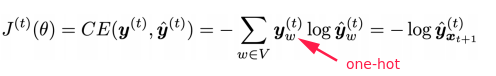• averaged for all steps t: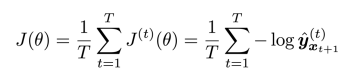in practice: compute t for entire corpus is too expensive

⇒ only compute for a sentence or a document

SGD: only compute loss for a batch of sentences

#### backprop

pb: the weights W is repeatedly applied, how to compute the grad?Multivariable Chain Rule: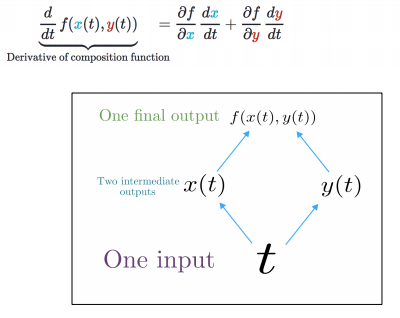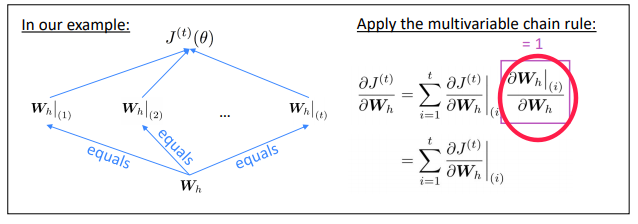#### backprop through time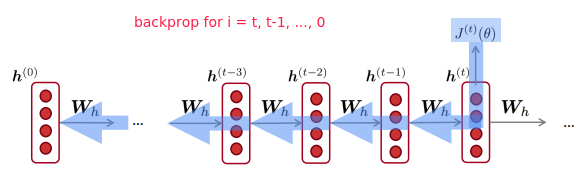## RNN Text Generation

use the RNN LM to gen text: sampled output is next step’s input.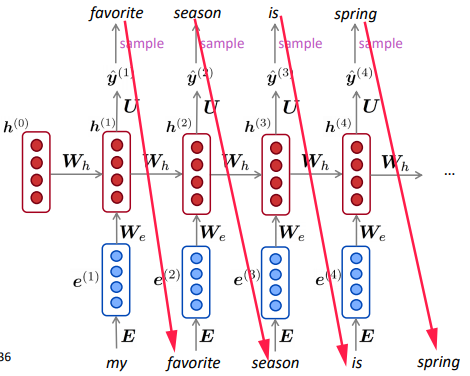## Evaluating Language Models

Perplexity: standard eval metrics of language models.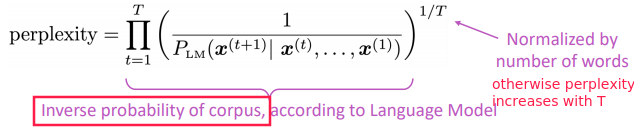Perplexity equals `exp(J(theta))` where J is cross-entropy loss ⇒ the smaller the better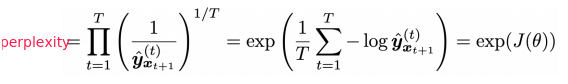Why should we care about Language Modeling?

• LM is a benchmark task that helps us measure our progress on understanding language
• LM is a subcomponent of many NLP tasks

## Recap

• Language model: system that predicts the next word
• Recurrent Neural Network:
• seq input of any length
• same weight on each step
• optionally produce output on each step

RNN can be used for LM, but can be used for other taskes.

• RNN for POS tagging: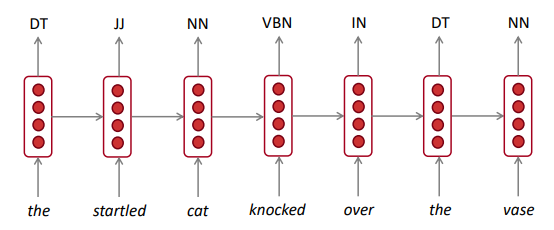• RNN for sentiment classification: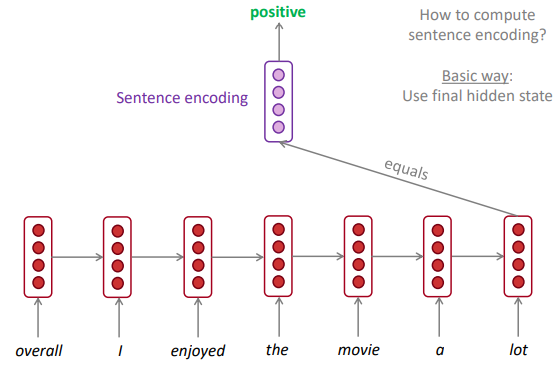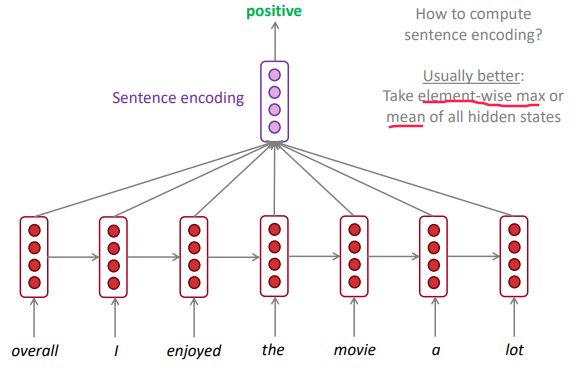• RNN as an encoder module: e.g.question answering, machine translation, etc.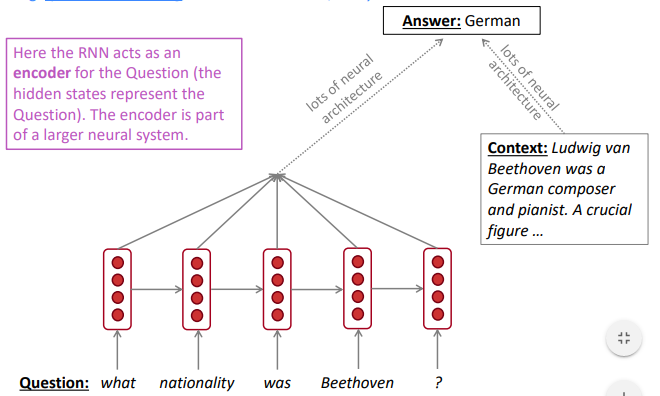• RNN used to gen text: e.g.speech recognition, machine translation, summarization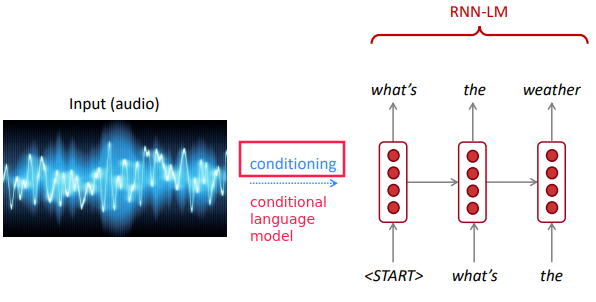terminology: vanilla RNN, GRU, LSTM, etc.